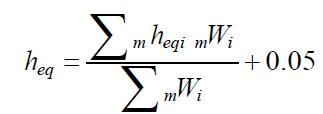Foundation, Concrete and Earthquake Engineering

### Equivalent Damping Ratio of Equivalent Single-Degree- of-Freedom (ESDOF) System

The equivalent damping ratio is defined by the viscous damping, hysteretic dissipation energy, elastic strain energy of a building and the radiation damping effects of the ground.

The equivalent damping ratio for the first mode is prescribed to be 0.05 at the damage- limitation limit state because the behavior of a building is basically elastic.

The equivalent viscous damping ratio at the life-safety limit state is defined by equating the energy dissipated by hysteretic behavior of a nonlinear system and the energy dissipated by viscous damping under stationary vibration in resonance. The equivalent damping ratio of an equivalent single-degree- of-freedom (ESDOF) system, stheq, is defined as follows (see Fig. 1).Fig 1 : Equivalent damping ratio using hysteretic energy dissipation
where,

stheq : equivalent damping ratio of an ESDOF system under resonant stationary vibration,

ΔW : dissipation energy of an ESDOF system, and,

W : potential energy of an ESDOF system (1Qb·1Δ/2).

The dissipation energy of a stationary hysteretic loop at the assumed maximum response of a building is either estimated by calculating the area of the supposed cyclic loop of the building in the nonlinear pushover analysis, or determined based on the equivalent damping ratio of each structural element considered.

Equation (1) does not hold in the response under nonstationary excitations such as earthquake motions. The equivalent damping ratio under stationary vibration must be reduced to correlate the maximum response of an equivalent linear system and a nonlinear system under earthquake motions. According to the analytical results, the equivalent damping ratio is reduced to approximately 80 percent of that calculated by Eq. (1).

The equivalent damping ratio, heq, of an ESDOF system should be in principle estimated as the weighted average with respect to strain energy of each member according to the provision:..........................(2)
where,

heq : equivalent damping ratio of an ESDOF system,

mheqi : equivalent damping ratio of member i , and,

mWi : strain energy stored in member i at ultimate deformation.

The equivalent damping ratio, mheqi, of member i is estimated as follows:

where,

μ: ductility factor of a member reached at the ultimate state of a building.

The factor of γ is the reduction factor considering the damping effect for the transitional seismic response of the building. It takes the values of 0.25 for ductile members and 0.2 for non-ductile ones. When all structural members of a building structure have the same hysteretic characteristics, the equivalent damping ratio of a whole building can be estimated by Eq. (3).